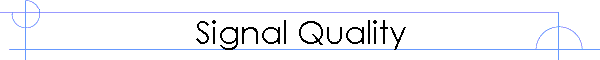Ultrasonics Applications and Solutions for Industry and Research

# Signal to Noise Ratio and Sensitivity

### Signal to Noise Ratio

The only practical measure of the utility of an ultrasonic system (or any other sensor) is the effective signal to noise ratio.  The signal to noise ratio is a measure of the relative size of a signal to the noise background.  For any practical measurement the signal to noise ratio (across a range of frequencies) determines how useful an ultrasonic system will be.  It is not possible to assign a signal to noise ratio to an isolated transducer or an ultrasonic system without specifying the exact configuration, test equipment, cables and conditions.  The ratios must be measured for each specific case.

Transducer manufacturers often refer to very high gain values for a particular transducer.  The gain is meant to relate the voltage produced when a sound wave of known pressure hits the front surface of the transducer.  But is a transducers gain sufficient to be able to compare two different transducers?  The short answer is that the gain alone is not enough information.  To provide an analogy, specifying just the gain is like being asked how long does it take to drive from New York to Chicago and answering 65 miles per hour!  It’s an incomplete answer.

Transducer gain characteristics and dynamic range specifications by themselves are just not enough information to know how a given system will perform.  Manufacturers sometimes specify noise thresholds.  Unfortunately these parameters, if taken out of context, are misleading.

There are dozens of factors that determine the effective dynamic range of any given ultrasonic system.  The noise performance of the preamplifiers, the electrical matching network, the cabling and connectors and the signal processing methods all play very important roles.

### Understanding the Signal to Noise Ratio

What does the signal to noise ratio describe?  In simple terms it is a measure of how large the signal is, relative to the background noise floor of the combined system.  The ratio is almost always expressed in dB so a value of 0.0 dB corresponds to the case where the signal and noise levels are equal.  A signal to noise ratio of 0.0 dB would mean that the signal would be difficult to detect and analyze because it is no larger than the surrounding noise.

The signal to noise ratio can also be negative.  A value of –20dB indicates that the signal level is 100 times smaller than the noise floor.  In this case the signal is below the noise floor.  Generally this would require changes to the setup to further reduce the noise levels or to increase signal levels.  A value of +20dB would indicate that the signal is 100 times larger than the noise floor.  Signal to noise ratios of +20dB or greater are considered to be ‘clean data’ that is easy to detect and analyze.

In engineering terms, there is always a trade-off between signal to noise ratio and the rate at which measurements can be made.  The actual rate at which measurements can be made and the achievable signal to noise ratio are strongly dependent on the particular system and transducers.  As a general guideline: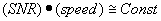For any given application there will be a minimum acceptable signal to noise ratio.  For a given ratio, there will be a maximum achievable speed.  This curve defines the envelope of the application and is useful to determine if a given application is practical for some particular purpose.

As an example, consider a scanning application where a set of transducers is used to make point measurements of a flat surface.  A technician setting up the system determines that a SNR of 20dB is achievable at a rate of 10 readings per second.  Increasing the signal to noise ratio to 26dB (which corresponds to doubling the signal to noise ratio) would require slowing the system down to 5 readings per second.  This trade-off in speed may or may not be acceptable depending on the application.

### Measuring the Signal to Noise Ratio

As an example, consider two capacitive transducers mounted vertically with a sample placed between the transducers.  The transducers have been aligned with the sample surface such that the signals through the sample are maximized.  Once the signal parameters have been established (i.e. frequency, duration, bandwidth and amplitude as well as the signal averaging), what is the effective signal to noise ratio of the setup?

When measuring signal strength, it is important to select a measurement tool that will cover the same time period regardless of the presence or absence of a signal.  The measurement consists of determining the total signal strength through the test sample and then the background signal level when the ultrasonic signal is completely obstructed.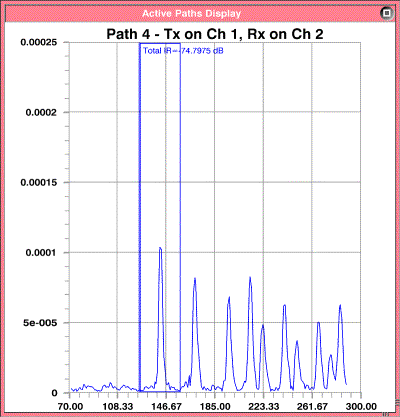Figure 1 Signal through 32 mm thick polystyrene using VN Instruments CAP3 transducers.  The particular setup happens to operate at 60 readings per second.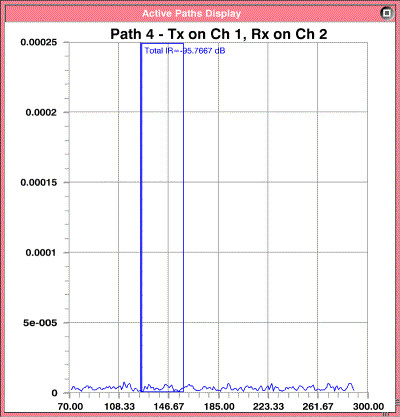Figure 2 Same as previous figure but with additional material used to obstruct the ultrasonic signal completely.

The SIA-7 has a measurement tool called the Total Integrated Response gate.  This tool measures the total energy found in a specified time window.  The tool even provides the data in dB form which makes the subsequent calculations very simple.  The SIA-7 Users Manual describes how to set up the gate and how it works.  Measurements of Total IR and other related parameters are best done using the QC tool.  This is a convenient and accurate means of determining the signal strength.  First consider the signal in air with no sample present.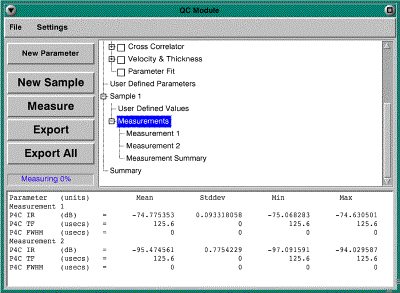Figure 3 The SIA-7 QC Module is a useful tool for making statistical measurements of samples.  In this case the values listed for Measurement 1 are –74.8 dB (taken with the sample in place).  The values taken for measurement 2 are –95.5 dB (with the ultrasonic signal completely obstructed).

The signal to noise ratio is simply the difference between these two values or 20.7dB.

Home ] Up ]